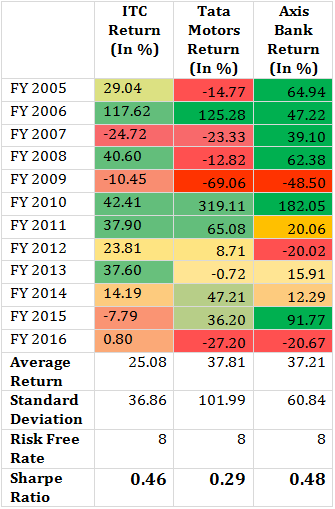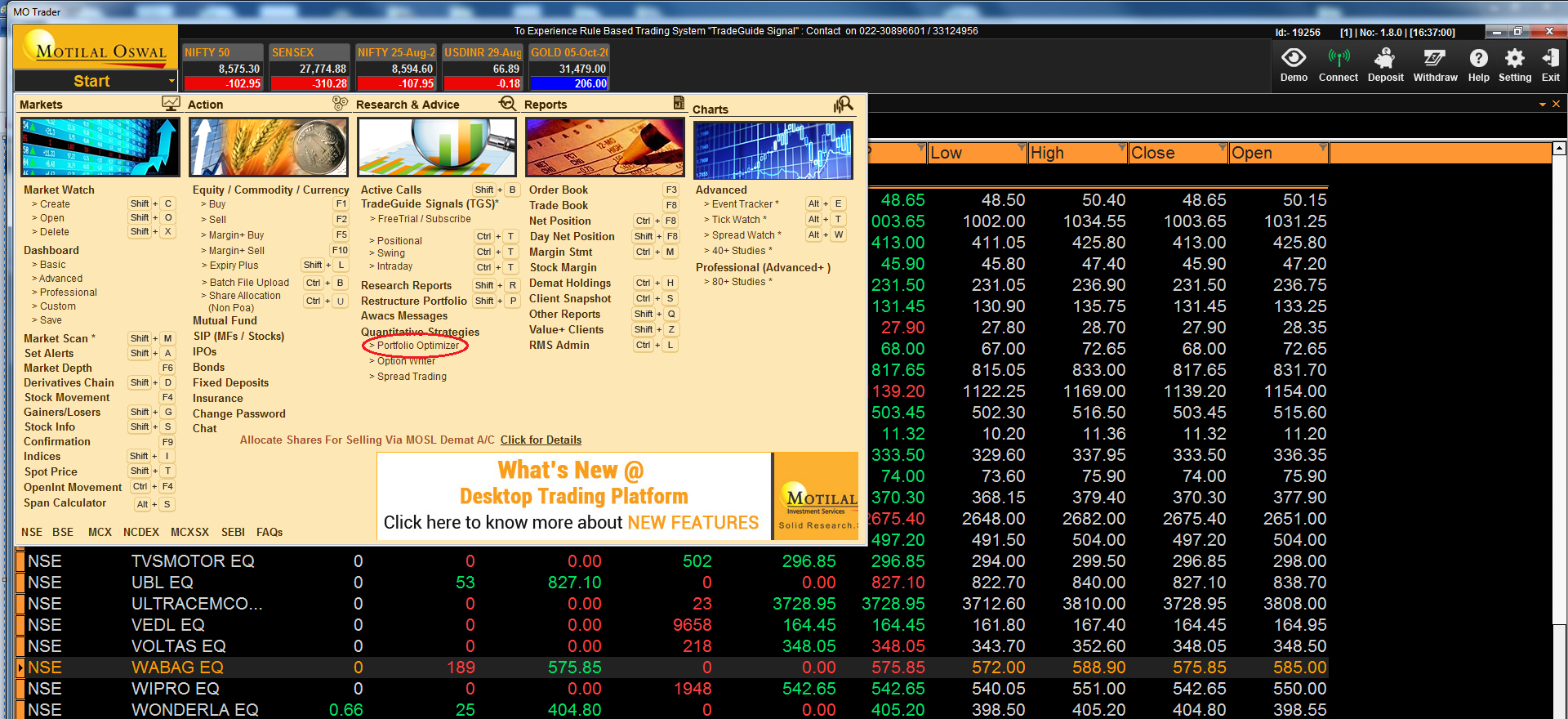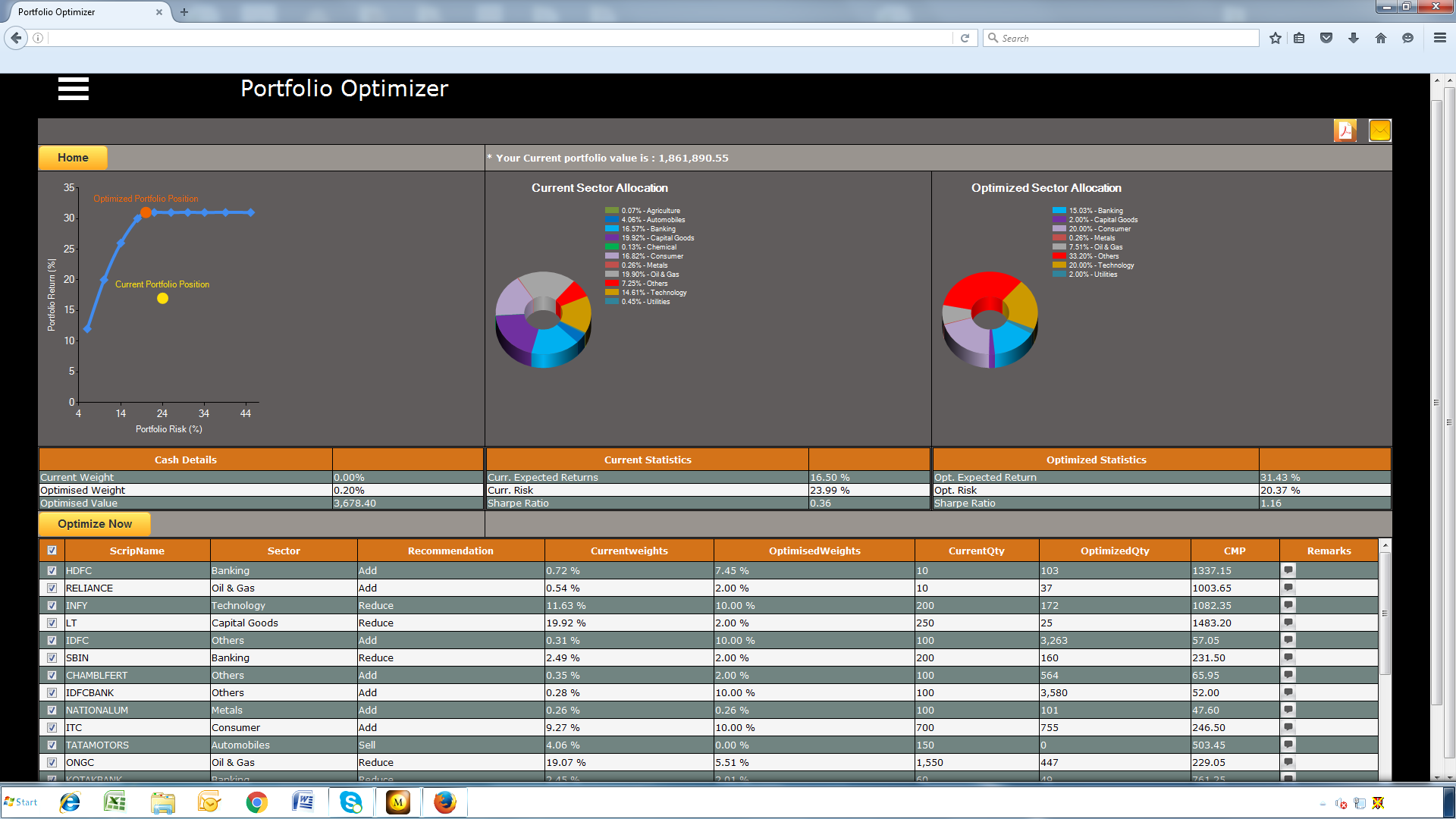Today, I will try to answer 2 questions that I’ve been asked more than a few times:

1. How should you rebalance your portfolio to replace underperforming stocks?
2. How frequently should you rebalance your portfolio?

Naturally, the answer to both these questions will depend upon many things most importantly – risk profile of the client and the portfolio.

The Justification for Portfolio Rebalancing

The reason why you should rebalance your portfolio (in 100% cases) is ‘Risk adjustment’. The idea should be to protect your portfolio from deteriorating by reducing risky assets depending on sectoral allocations.

This is particularly important for investors who do not follow a top down approach, since they are more susceptible to picking many stocks in high risk sectors and may ignore certainty of return, where “certain returns” are not as attractive as in case of riskier investments.

Add to this, the loss averse nature of most investors and the result is continuous deterioration in portfolio value.

For starters, follow a top down approach and divide your holdings in stocks belonging to different sectors giving higher weight-age to core/ well established sectors that are not facing any extraordinary headwinds.

Once you have zeroed down on allocations in various sectors, the next step is to allocate the investment amount in stocks within these sectors. This is where it may be worthwhile to take the help of a tool which allocates money on the basis of a risk-reward using an optimising tool based on Sharpe Ratio.

## How to Rebalance Your Portfolio Using the Sharpe Ratio?

The simplest strategy to allocate stocks is to divide your investment amount equally between the stocks you have chosen to invest in. This can be the simplest method but lacks the ability to use synergies that might be present in your portfolio. There are various techniques which are used for portfolio rebalancing. Below, I will discuss how you can use Sharpe ratio to rebalance your portfolio.

What is Sharpe Ratio?

In simple terms, Sharpe ratio is a measure of reward to risk. Higher the Sharpe ratio value, better the investment from a reward/risk perspective.

BELOW, I WILL GIVE YOU A TOOL TO USE SHARPE RATIO TO REBALANCE YOUR PORTFOLIO AUTOMATICALLY.

How to Calculate Sharpe Ratio?

To calculate the Sharpe Ratio you need a stock’s Expected Return and Standard Deviation. You also need the best risk-free rate.

The Sharpe Ratio calculation is:

S(x)  =  (Rx – Rf) / Std Dev(x)

Where:

x is an investment (e.g. ITC)

Rx is the average annual rate of return of x

Rf is the best available rate of return of a “risk-free” security (i.e. Fixed Deposit)

And Std Dev(x) is the standard deviation of Rx.

Once you’ve calculated the Sharpe Ratio for each stock in your portfolio, you can use a simple method to allocate your stocks more intelligently than just throwing equal amounts of cash at your stocks.

### Steps to Rebalance your Portfolio:

1. Compute the Sharpe Ratio for each stock in your portfolio (using the above formula).
2. Sum all of the Sharpe Ratio values.
3. Divide each stock’s Sharpe Ratio by the sum of the Sharpe Ratios. This gives the percentage allocation for that stock.

Example: Let’s say, you have 3 stocks in your portfolio – ITC, Tata Motors, Axis Bank.

First Step – Calculate Sharpe Ratio of Each StockSecond Step – Sum all of the Sharpe Ratio values.Stock Sharpe Ratio ITC 0.46 Tata Motors 0.29 NTPC 0.48 Sharpe Ratio Sum 1.23 In this case, the sum of the Sharpe Ratios is 1.23.

Third Step – Divide the Sharpe Ratio of ITC by the sum of 1.23 to get:

0.46 / 1.23 = 0.3739837 (or about 37%).

Do the same for Tata Motors and Axis Bank, and you’ll get 0.2357724 (approximately 24%) and 0.3902439 (39%) respectively.

So, your rebalanced portfolio allocation will be: ITC = 37%, Tata Motors = 24% and Axis Bank = 39%.

____________________

A Tool for Trading members

The advantage of this strategy is that the better reward-to-risk stocks get a larger share of your investment funds. If you’d rather not do these calculations manually, try the portfolio optimizer function in our trading terminal. Here’s a step by step guide:

Note: You will need to have your stock portfolio that you need to optimize in your trading terminal.

In Orion Lite follow the steps below:

`Click on images to enlarge`

Step 1 – Click on Portfolio Optimizer.Step 2 – You will see your portfolio here. Select Best Sharpe Ratio and Press Submit.Step 3 – You will See Your Optimized/Suggested Allocations. Click on Optimize Now.